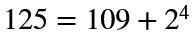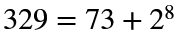# Problem 57869. Identify de Polignac numbers

The numbers 125 and 329 can be written as the sum of a prime and a power of 2. For example,, and. The numbers 127 and 331, which are examples of de Polignac numbers, cannot be written in this way.
Write a function that determines whether an odd number is a de Polignac number.

### Solution Stats

42.42% Correct | 57.58% Incorrect
Last Solution submitted on May 15, 2023

### Community Treasure Hunt

Find the treasures in MATLAB Central and discover how the community can help you!

Start Hunting!